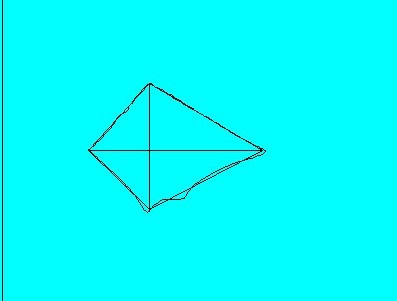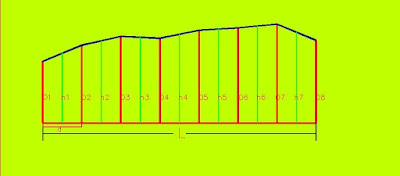HOW TO CALCULATE AREA IN THE MAP

# HOW TO CALCULATE AREA IN THE MAP

HOW TO CALCULATE AREA IN THE  MAP
• INTRODUCTION
AREA:-Area is defined as tract the area of land in the horizontal plane. All types of plan/map is drawn in a horizontal plane then calculate area in a horizontal plane.AREA

The units of area is hectare and square meter.

•  There are generally three methods use for calculate area in the map.
I Graphical method
ii  Calculation method
iii  Instrumental method
iv  Software method
•   i.  Graphical method:-
(a) By division into triangles. In this method the figure is divided into a number of The base and height of the triangles are measured with a scale. The irregular boundary is adjusted by the give and take or equalizing method. In fig: 1fig:-1

(b) By Division into squares:- In this method, the area is calculated by the use of tracing paper.          In the tracing paper no, of squares drawn on the tracing paper. Each square represents in square meter or square centimetre. The tracing paper placed on the drawing and counted no. of the whole The portion of the square which covered boundary estimate as a whole square.
Then the area = total no of squares * square meter of one square (in the tracing paper)  Shown          in fig:-2

•  ii. Calculation method:-  In this method area calculate by a baseline taken through the area and divided into a number of equal parts. The ordinate at each of the points of division are drawn and scaled.
( c)  The mid - ordinate rule:- In this method the ordinates are measured at the mid-points of               each  division and the area is calculated by the formulafig 3
In the fig:-3
O1, O2, O3..............O8 are the ordinates at an equal interval.
h1,h2,h3................h7 are the mid ordinates.
d=  common distance between ordinates.
L= Length of baseline.
Area of the plot. h1*d+h2*d+h3*d+.................h7*d
Area = (h1+ h2+ h3+..........h7)*d

(b) The Average ordinate rule- In this method, the ordinates are drawn and scaled at each of the points of divisions of the base. The average of these ordinates multiplied by the length of the base line gives the required area. fig 4fig 4

O1, O2, O3..............O6 are the ordinates at an equal interval.
L= Length of baseline.
n= no. of divisions.
n+1=no. of ordinates.
Area= O1+O2+O3+.......O6/On+1*L
Area=sum of ordinates*length of the baseline/no.of ordinates.

(c) The Trapezoidal Rule:- In this method the no. of ordinates are draw at a commonand the ordinates are considered trapezoids. The total area is divided into no. of trapezoids.

O1, O2, O3..............O6 are the ordinates at an equal interval.
d=  common distance between ordinates.
Area= Common distance/2{(1st ordinate+last ordinate+2(sum of other ordinate)}
This method used where if the division is even

Area= d/3 { (1st ordinate+last ordinate)+4( sum of even ordinate)+2(odd ordinate)

• iii Instrument method:- In this method, the area is calculated from the use of or digital planimeters. It more accurate method and gives the result within one per cent error.

•  iv method:-  In this method, the area is computed from the users of the software. In the software, the figure is drawn in digital form from survey data or scan from the image object. The software gives direct area.